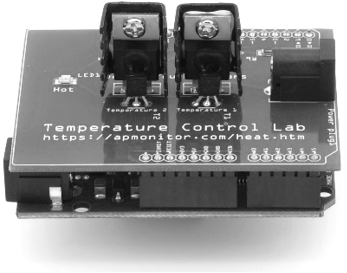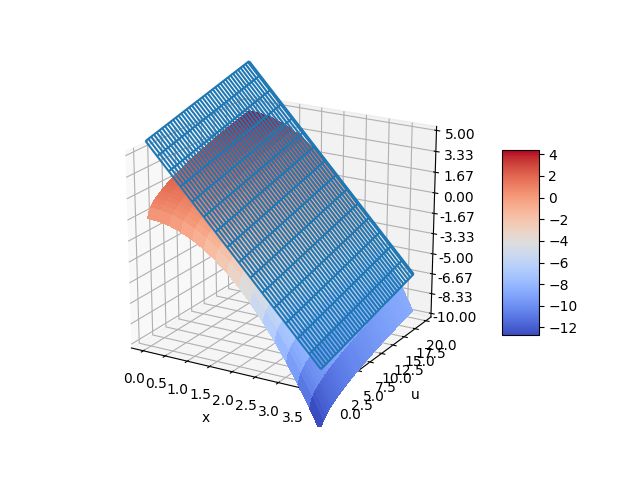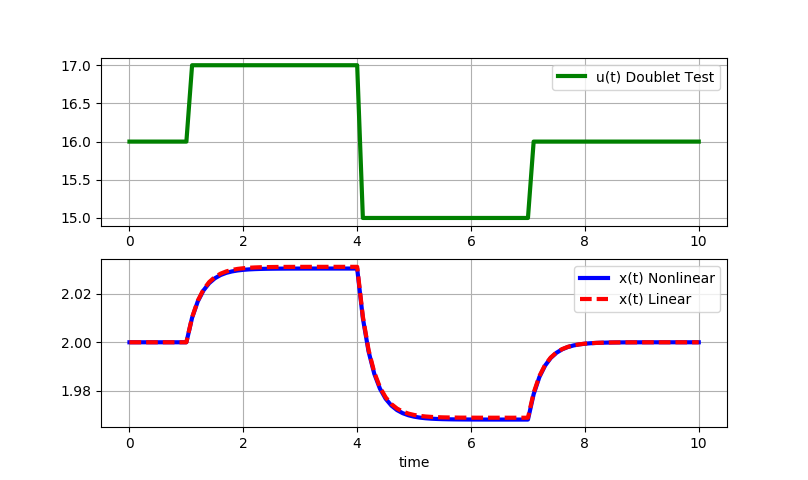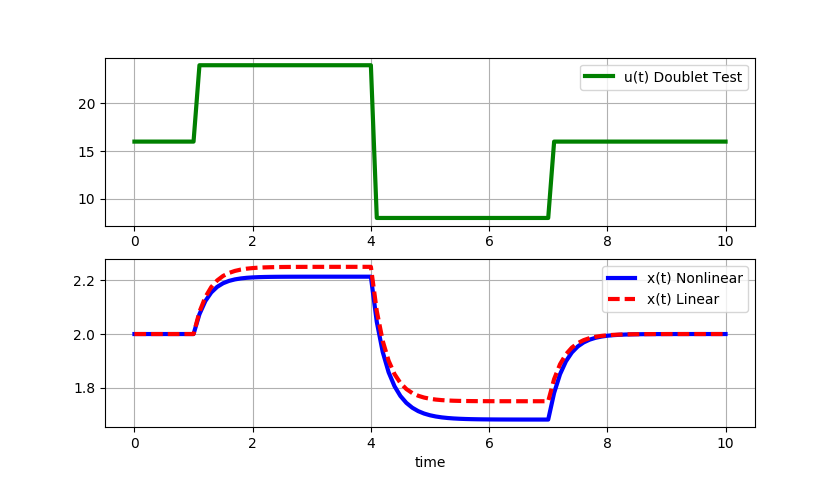## Linearization of Differential EquationsLinearization is the process of taking the gradient of a nonlinear function with respect to all variables and creating a linear representation at that point. It is required for certain types of analysis such as stability analysis, solution with a Laplace transform, and to put the model into linear state-space form. Consider a nonlinear differential equation model that is derived from balance equations with input u and output y.

$$\frac{dy}{dt} = f(y,u)$$

The right hand side of the equation is linearized by a Taylor series expansion, using only the first two terms.

$$\frac{dy}{dt} = f(y,u) \approx f \left(\bar y, \bar u\right) + \frac{\partial f}{\partial y}\bigg|_{\bar y,\bar u} \left(y-\bar y\right) + \frac{\partial f}{\partial u}\bigg|_{\bar y,\bar u} \left(u-\bar u\right)$$

If the values of \bar u and \bar y are chosen at steady state conditions then f(\bar y, \bar u)=0 because the derivative term {dy}/{du}=0 at steady state. To simplify the final linearized expression, deviation variables are defined as y' = y-\bar y and u' = u - \bar u. A deviation variable is a change from the nominal steady state conditions. The derivatives of the deviation variable is defined as {dy'}/{dt} = {dy}/{dt} because {d\bar y}/{dt} = 0 in {dy'}/{dt} = {d(y-\bar y)}/{dt} = {dy}/{dt} - \cancel{{d\bar y}/{dt}}. If there are additional variables such as a disturbance variable d then it is added as another term in deviation variable form d' = d - \bar d.

$$\frac{dy'}{dt} = \alpha y' + \beta u' + \gamma d'$$

The values of the constants \alpha, \beta, and \gamma are the partial derivatives of f(y,u,d) evaluated at steady state conditions.

$$\alpha = \frac{\partial f}{\partial y}\bigg|_{\bar y,\bar u,\bar d} \quad \quad \beta = \frac{\partial f}{\partial u}\bigg|_{\bar y,\bar u,\bar d} \quad \quad \gamma = \frac{\partial f}{\partial d}\bigg|_{\bar y,\bar u,\bar d}$$

#### Example

Part A: Linearize the following differential equation with an input value of u=16.

$$\frac{dx}{dt} = -x^2 + \sqrt{u}$$

Part B: Determine the steady state value of x from the input value and simplify the linearized differential equation.

Part C: Simulate a doublet test with the nonlinear and linear models and comment on the suitability of the linear model to represent the original nonlinear equation solution.

Part A Solution: The equation is linearized by taking the partial derivative of the right hand side of the equation for both x and u.

$$\frac{\partial \left(-x^2 + \sqrt{u}\right)}{\partial x} = \alpha = -2 \, x$$

$$\frac{\partial \left(-x^2 + \sqrt{u}\right)}{\partial u} = \beta = \frac{1}{2} \frac{1}{\sqrt{u}}$$

The linearized differential equation that approximates \frac{dx}{dt}=f(x,u) is the following:

$$\frac{dx}{dt} = f \left(x_{ss}, u_{ss}\right) + \frac{\partial f}{\partial x}\bigg|_{x_{ss},u_{ss}} \left(x-x_{ss}\right) + \frac{\partial f}{\partial u}\bigg|_{x_{ss},u_{ss}} \left(u-u_{ss}\right)$$

Substituting in the partial derivatives results in the following differential equation:

$$\frac{dx}{dt} = 0 + \left(-2 x_{ss}\right) \left(x-x_{ss}\right) + \left(\frac{1}{2} \frac{1}{\sqrt{u_{ss}}}\right) \left(u-u_{ss}\right)$$

This is further simplified by defining new deviation variables as x' = x - x_{ss} and u' = u - u_{ss}.

$$\frac{dx'}{dt} = \alpha x' + \beta u'$$

Part B Solution: The steady state values are determined by setting \frac{dx}{dt}=0 and solving for x.

$$0 = -x_{ss}^2 + \sqrt{u_{ss}}$$

$$x_{ss}^2 = \sqrt{16}$$

$$x_{ss} = 2$$

At steady state conditions, frac{dx}{dt}=0 so f (x_{ss}, u_{ss})=0 as well. Plugging in numeric values gives the simplified linear differential equation:

$$\frac{dx}{dt} = -4 \left(x-2\right) + \frac{1}{8} \left(u-16\right)$$

The partial derivatives can also be obtained from Python, either symbolically with SymPy or else numerically with SciPy.

# analytic solution with Python
import sympy as sp
sp.init_printing()
# define symbols
x,u = sp.symbols(['x','u'])
# define equation
dxdt = -x**2 + sp.sqrt(u)

print(sp.diff(dxdt,x))
print(sp.diff(dxdt,u))

# numeric solution with Python
import numpy as np
from scipy.misc import derivative
u = 16.0
x = 2.0
def pd_x(x):
dxdt = -x**2 + np.sqrt(u)
return dxdt
def pd_u(u):
dxdt = -x**2 + np.sqrt(u)
return dxdt

print('Approximate Partial Derivatives')
print(derivative(pd_x,x,dx=1e-4))
print(derivative(pd_u,u,dx=1e-4))

print('Exact Partial Derivatives')
print(-2.0*x) # exact d(f(x,u))/dx
print(0.5 / np.sqrt(u)) # exact d(f(x,u))/du

Python program results

 -2*x
1/(2*sqrt(u))
Approximate Partial Derivatives
-4.0
0.125
Exact Partial Derivatives
-4.0
0.125


The nonlinear function for \frac{dx}{dt} can also be visualized with a 3D contour map. The choice of steady state conditions x_{ss} and u_{ss} produces a planar linear model that represents the nonlinear model only at a certain point. The linear model can deviate from the nonlinear model if used further away from the conditions at which the linear model is derived.from mpl_toolkits.mplot3d import Axes3D
import matplotlib.pyplot as plt
from matplotlib import cm
from matplotlib.ticker import LinearLocator, FormatStrFormatter
import numpy as np

fig = plt.figure()
ax = fig.gca(projection='3d')

# Make data.
X = np.arange(0, 4, 0.25)
U = np.arange(0, 20, 0.25)
X, U = np.meshgrid(X, U)
DXDT = -X**2 + np.sqrt(U)
LIN = -4.0 * (X-2.0) + 1.0/8.0 * (U-16.0)

# Plot the surface.
surf = ax.plot_wireframe(X, U, LIN)
surf = ax.plot_surface(X, U, DXDT, cmap=cm.coolwarm,
linewidth=0, antialiased=False)

# Customize the z axis.
ax.set_zlim(-10.0, 5.0)
ax.zaxis.set_major_locator(LinearLocator(10))
ax.zaxis.set_major_formatter(FormatStrFormatter('%.02f'))

# Add a color bar which maps values to colors.
fig.colorbar(surf, shrink=0.5, aspect=5)

plt.xlabel('x')
plt.ylabel('u')

plt.show()

Part C Solution: The final step is to simulate a doublet test with the nonlinear and linear models.

Small step changes (+/-1): Small step changes in u lead to nearly identical responses for the linear and nonlinear solutions. The linearized model is locally accurate.Large step changes (+/-8): As the magnitude of the doublet steps increase, the linear model deviates further from the original nonlinear equation solution.import numpy as np
from scipy.integrate import odeint
import matplotlib.pyplot as plt

# function that returns dz/dt
def model(z,t,u):
x1 = z
x2 = z
dx1dt = -x1**2 + np.sqrt(u)
dx2dt = -4.0*(x2-2.0) + (1.0/8.0)*(u-16.0)
dzdt = [dx1dt,dx2dt]
return dzdt

x_ss = 2.0
u_ss = 16.0

# initial condition
z0 = [x_ss,x_ss]

# final time
tf = 10

# number of time points
n = tf * 10 + 1

# time points
t = np.linspace(0,tf,n)

# step input
u = np.ones(n) * u_ss
# magnitude of step
m = 8.0
# change up m at time = 1.0
u[11:] = u[11:] + m
# change down 2*m at time = 4.0
u[41:] = u[41:] - 2.0 * m
# change up m at time = 7.0
u[71:] = u[71:] + m

# store solution
x1 = np.empty_like(t)
x2 = np.empty_like(t)
# record initial conditions
x1 = z0
x2 = z0

# solve ODE
for i in range(1,n):
# span for next time step
tspan = [t[i-1],t[i]]
# solve for next step
z = odeint(model,z0,tspan,args=(u[i],))
# store solution for plotting
x1[i] = z
x2[i] = z
# next initial condition
z0 = z

# plot results
plt.figure(1)
plt.subplot(2,1,1)
plt.plot(t,u,'g-',linewidth=3,label='u(t) Doublet Test')
plt.grid()
plt.legend(loc='best')
plt.subplot(2,1,2)
plt.plot(t,x1,'b-',linewidth=3,label='x(t) Nonlinear')
plt.plot(t,x2,'r--',linewidth=3,label='x(t) Linear')
plt.xlabel('time')
plt.grid()
plt.legend(loc='best')
plt.show()